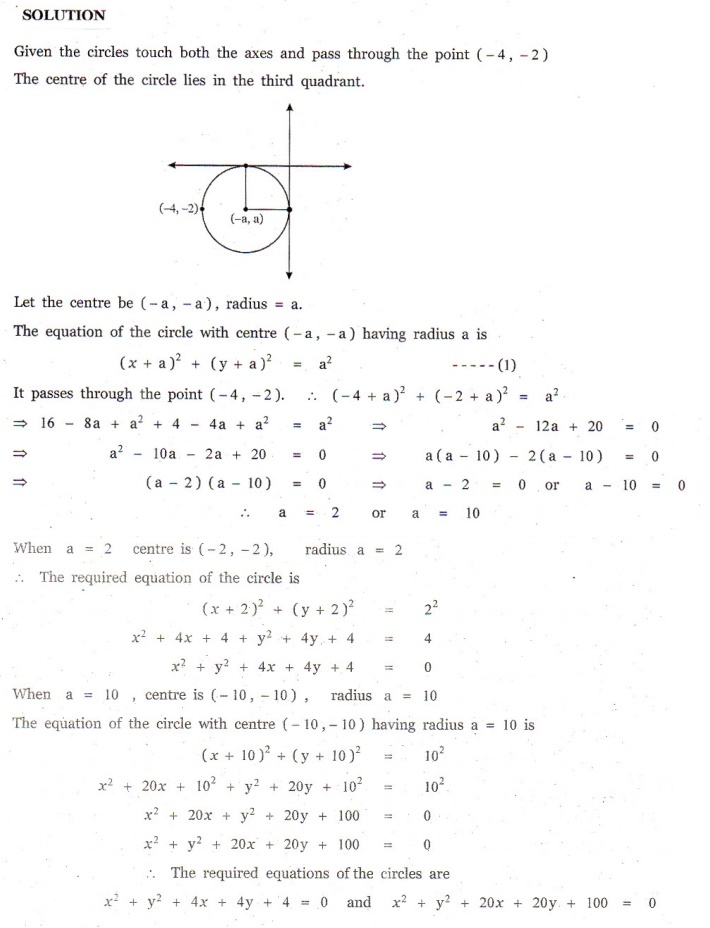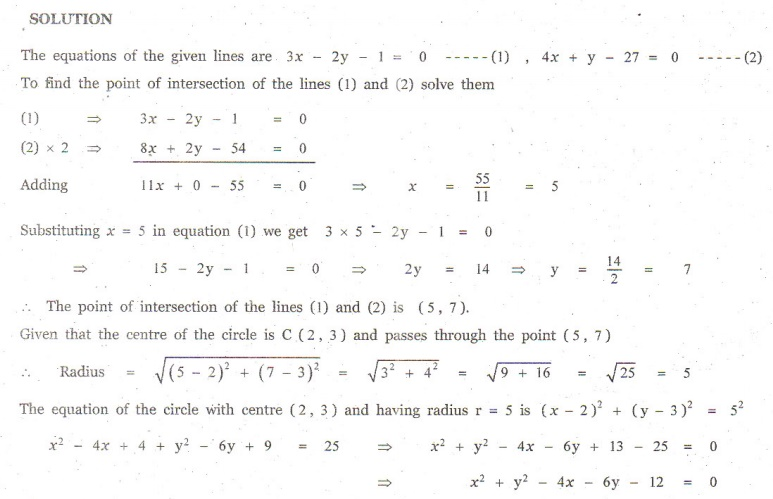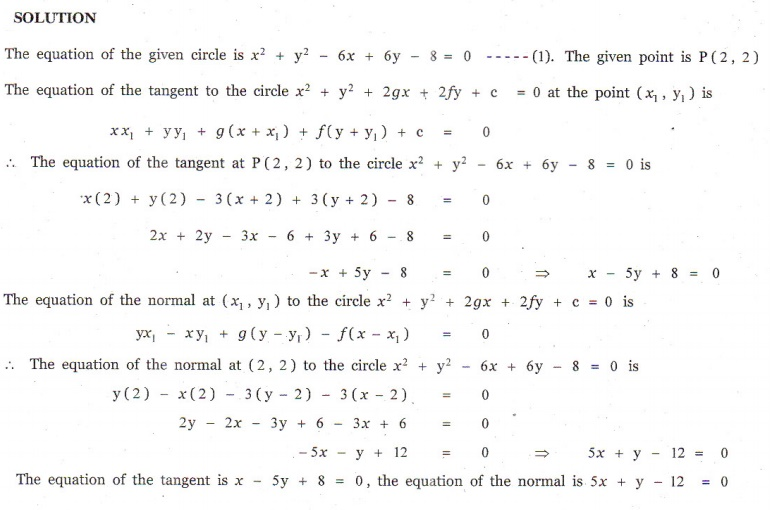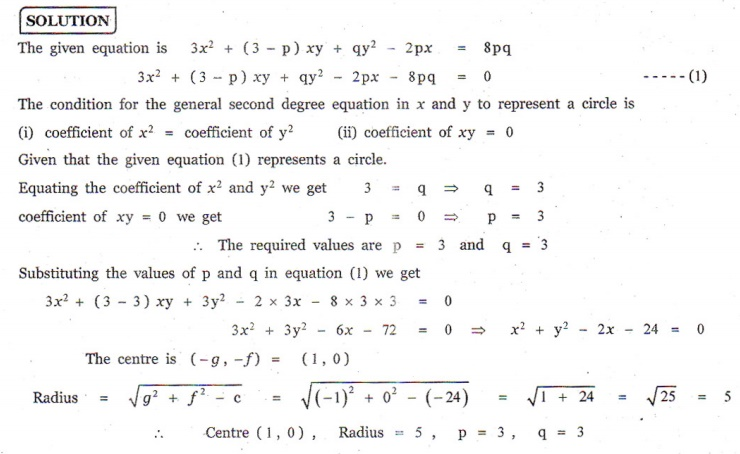Home | | Maths 12th Std | Exercise 5.1: Circle

# Exercise 5.1: Circle

Equation of a circle in standard form, Equations of tangent and normal at a point P on a given circle, Condition for the line y = mx + c to be a tangent to the circle and finding the point of contact - Maths Book back answers and solution for Exercise questions

EXERCISE 5.1

1. Obtain the equation of the circles with radius 5 cm and touching x-axis at the origin in general form.2. Find the equation of the circle with centre (2, 1) and passing through the point (3, 6) in standard form.3. Find the equation of circles that touch both the axes and pass through (4, 2) in general form.4. Find the equation of the circle with centre (2, 3) and passing through the intersection of the lines 3 2 = 0 and 4+  27 = 0 .5. Obtain the equation of the circle for which (3, 4) and (2, 7) are the ends of a diameter.6. Find the equation of the circle through the points (1, 0), (1, 0) , and (0,1) .7. A circle of area 9π square units has two of its diameters along the lines + = 5 and  = 1. Find the equation of the circle.8. If = 2√2+ is a tangent to the circle x+ y= 16 , find the value of .9. Find the equation of the tangent and normal to the circle x+ y 6+ 6  8 = 0 at (2, 2) .10. Determine whether the points (2,1), (0, 0) and (4, 3) lie outside, on or inside the circle x+ y 5+ 2 − 5 = 0 .11. Find centre and radius of the following circles.

(i)  x2  + ( y + 2)2  = 0

(ii)  x2  + y2  + 6x - 4 y + 4 = 0

(iii) x2 + y2 - x + 2 y - 3 = 0

(iv) 2x2 + 2 y2  6x + 4 y + 2 = 012. If the equation 3x+ (3 − pxy qy− 2 px = 8 pq represents a circle, find and . Also determine the centre and radius of the circle.